## STAM101 :: Lecture 20 :: 2cube factorial experiments in RBD – lay out – analysis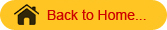2cube Factorial Experiment in RBD
2cube  factorial experiment mean three factors each at two levels. Suppose the three factors are A, B and C are tried with two levels the total number of combinations will be eight i.e. a0b0c0, a0b0c1, a0b1c0, a0b1c1, a1b0c0, a1b0c1, a1b1c0 and a1b1c1.
The allotment of these eight treatment combinations will be as allotted in RBD. That is each block is divided into eight experimental units. By using the random numbers these eight combinations are allotted at random for each block separately.
The analysis of variance table for three factors A with a levels, B with b levels and C with c levels with r replications tried in RBD will be as follows:

 Sources of Variation d.f. SS MS F Replications r-1 RSS RMS Factor A a-1 ASS AMS AMS / EMS Factor B b-1 BSS BMS BMS / EMS Factor C c-1 CSS CMS CMS / EMS AB (a-1)(b-1) ABSS ABMS ABMS / EMS AC (a-1)(c-1) ACSS ACMS ACMS / EMS BC (b-1)(c-1) BCSS BCMS BCMS / EMS ABC (a-1)(b-1)(c-1) ABCSS ABCMS ABCMS / EMS Error (r-1)(abc-1) ESS EMS Total rabc-1 TSS

Analysis

1. Arrange the results as per treatment combinations and replications.
 Treatment combination Replication R1                 R2                      R3                … Treatment Total a0b0c0 T1 a0b0c1 T2 a0b1c0 T3 a0b1c1 T4 a1b0c0 T5 a1b0c1 T6 a1b1c0 T7 a1b1c1 T8

As in the previous designs calculate the replication totals to calculate the CF, RSS, TSS, overall TrSS in the usual way. To calculate ASS, BSS, CSS, ABSS, ACSS, BCSS and ABCSS, form three two way tables A X B, AXC and BXC.
AXB two way table can be formed by taking the levels of A in rows and levels of B in the columns.  To get the values in this table the missing factor is replication. That is by adding over replication we can form this table.
A X B Two way table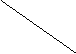B                   A b0 b1 Total a0 a0 b0 a0 b1 A0 a1 a1 b0 a1 b1 A1 Total B0 B1 Grand Total

ASS=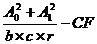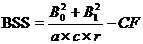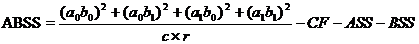A X C two way table can be formed by taking the levels of A in rows and levels of C in the columns
A X C Two way tableC                   A c0 c1 Total a0 a0 c0 a0 c1 A0 a1 a1 c0 a1 c1 A1 Total C0 C1 Grand Total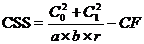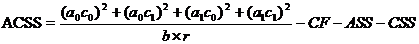B X C two way table can be formed by taking the levels of B in rows and levels of C in the columns
B X C Two way table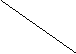C                   B c0 c1 Total b0 b0 c0 b0 c1 B0 b1 b1 c0 b1 c1 B1 Total C0 C1 Grand Total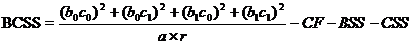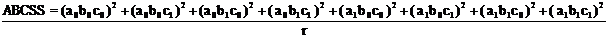-CF-ASS-BSS-CSS-ABSS-ACSS-BCSS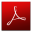Download this lecture as PDF here